# Dial’s Algorithm (Optimized Dijkstra for small range weights)

Dijkstra’s shortest path algorithm runs in O(Elog V) time when implemented with adjacency list representation (See C implementation and STL based C++ implementations for details).

```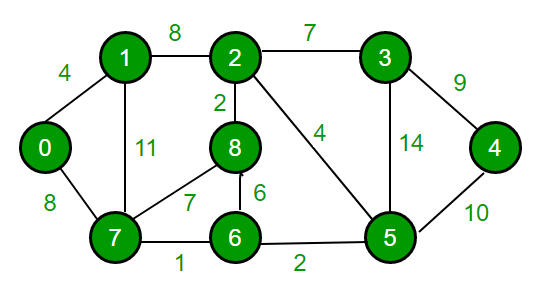Input : Source = 0, Maximum Weight W = 14
Output :
Vertex   Distance from Source
0                0
1                4
2                12
3                19
4                21
5                11
6                9
7                8
8                14```

Can we optimize Dijkstra’s shortest path algorithm to work better than O(E log V) if maximum weight is small (or range of edge weights is small)?
For example, in the above diagram, maximum weight is 14. Many a times the range of weights on edges in is in small range (i.e. all edge weight can be mapped to 0, 1, 2.. w where w is a small number). In that case, Dijkstra’s algorithm can be modified by using different data structure, buckets, which is called dial implementation of dijkstra’s algorithm. time complexity is O(E + WV) where W is maximum weight on any edge of graph, so we can see that, if W is small then this implementation runs much faster than traditional algorithm. Following are important observations.

• Maximum distance between any two node can be at max w(V – 1) (w is maximum edge weight and we can have at max V-1 edges between two vertices).
• In Dijkstra algorithm, distances are finalized in non-decreasing, i.e., distance of the closer (to given source) vertices is finalized before the distant vertices.

Algorithm

Below is complete algorithm:

1. Maintains some buckets, numbered 0, 1, 2,…,wV.
2. Bucket k contains all temporarily labeled nodes with distance equal to k.
3. Nodes in each bucket are represented by list of vertices.
4. Buckets 0, 1, 2,..wV are checked sequentially until the first non-empty bucket is found. Each node contained in the first non-empty bucket has the minimum distance label by definition.
5. One by one, these nodes with minimum distance label are permanently labeled and deleted from the bucket during the scanning process.
6. Thus operations involving vertex include:
• Checking if a bucket is empty
• Adding a vertex to a bucket
• Deleting a vertex from a bucket.
7. The position of a temporarily labeled vertex in the buckets is updated accordingly when the distance label of a vertex changes.
8. Process repeated until all vertices are permanently labeled (or distances of all vertices are finalized).

Implementation

Since the maximum distance can be w(V – 1), we create wV buckets (more for simplicity of code) for implementation of algorithm which can be large if w is big.

 `// C++ Program for Dijkstra's dial implementation ` `#include ` `using` `namespace` `std; ` `# define INF 0x3f3f3f3f ` ` `  `// This class represents a directed graph using ` `// adjacency list representation ` `class` `Graph ` `{ ` `    ``int` `V;  ``// No. of vertices ` ` `  `    ``// In a weighted graph, we need to store vertex ` `    ``// and weight pair for every edge ` `    ``list< pair<``int``, ``int``> > *adj; ` ` `  `public``: ` `    ``Graph(``int` `V);  ``// Constructor ` ` `  `    ``// function to add an edge to graph ` `    ``void` `addEdge(``int` `u, ``int` `v, ``int` `w); ` ` `  `    ``// prints shortest path from s ` `    ``void` `shortestPath(``int` `s, ``int` `W); ` `}; ` ` `  `// Allocates memory for adjacency list ` `Graph::Graph(``int` `V) ` `{ ` `    ``this``->V = V; ` `    ``adj = ``new` `list< pair<``int``, ``int``> >[V]; ` `} ` ` `  `//  adds edge between u and v of weight w ` `void` `Graph::addEdge(``int` `u, ``int` `v, ``int` `w) ` `{ ` `    ``adj[u].push_back(make_pair(v, w)); ` `    ``adj[v].push_back(make_pair(u, w)); ` `} ` ` `  `// Prints shortest paths from src to all other vertices. ` `// W is the maximum weight of an edge ` `void` `Graph::shortestPath(``int` `src, ``int` `W) ` `{ ` `    ``/* With each distance, iterator to that vertex in ` `       ``its bucket is stored so that vertex can be deleted ` `       ``in O(1) at time of updation. So ` `    ``dist[i].first = distance of ith vertex from src vertex ` `    ``dits[i].second = iterator to vertex i in bucket number */` `    ``vector::iterator> > dist(V); ` ` `  `    ``// Initialize all distances as infinite (INF) ` `    ``for` `(``int` `i = 0; i < V; i++) ` `        ``dist[i].first = INF; ` ` `  `    ``// Create buckets B[]. ` `    ``// B[i] keep vertex of distance label i ` `    ``list<``int``> B[W * V + 1]; ` ` `  `    ``B.push_back(src); ` `    ``dist[src].first = 0; ` ` `  `    ``// ` `    ``int` `idx = 0; ` `    ``while` `(1) ` `    ``{ ` `        ``// Go sequentially through buckets till one non-empty ` `        ``// bucket is found ` `        ``while` `(B[idx].size() == 0 && idx < W*V) ` `            ``idx++; ` ` `  `        ``// If all buckets are empty, we are done. ` `        ``if` `(idx == W * V) ` `            ``break``; ` ` `  `        ``// Take top vertex from bucket and pop it ` `        ``int` `u = B[idx].front(); ` `        ``B[idx].pop_front(); ` ` `  `        ``// Process all adjacents of extracted vertex 'u' and ` `        ``// update their distanced if required. ` `        ``for` `(``auto` `i = adj[u].begin(); i != adj[u].end(); ++i) ` `        ``{ ` `            ``int` `v = (*i).first; ` `            ``int` `weight = (*i).second; ` ` `  `            ``int` `du = dist[u].first; ` `            ``int` `dv = dist[v].first; ` ` `  `            ``// If there is shorted path to v through u. ` `            ``if` `(dv > du + weight) ` `            ``{ ` `                ``// If dv is not INF then it must be in B[dv] ` `                ``// bucket, so erase its entry using iterator ` `                ``// in O(1) ` `                ``if` `(dv != INF) ` `                    ``B[dv].erase(dist[v].second); ` ` `  `                ``//  updating the distance ` `                ``dist[v].first = du + weight; ` `                ``dv = dist[v].first; ` ` `  `                ``// pushing vertex v into updated distance's bucket ` `                ``B[dv].push_front(v); ` ` `  `                ``// storing updated iterator in dist[v].second ` `                ``dist[v].second = B[dv].begin(); ` `            ``} ` `        ``} ` `    ``} ` ` `  `    ``// Print shortest distances stored in dist[] ` `    ``printf``(``"Vertex   Distance from Source\n"``); ` `    ``for` `(``int` `i = 0; i < V; ++i) ` `        ``printf``(``"%d     %d\n"``, i, dist[i].first); ` `} ` ` `  `// Driver program to test methods of graph class ` `int` `main() ` `{ ` `    ``// create the graph given in above fugure ` `    ``int` `V = 9; ` `    ``Graph g(V); ` ` `  `    ``//  making above shown graph ` `    ``g.addEdge(0, 1, 4); ` `    ``g.addEdge(0, 7, 8); ` `    ``g.addEdge(1, 2, 8); ` `    ``g.addEdge(1, 7, 11); ` `    ``g.addEdge(2, 3, 7); ` `    ``g.addEdge(2, 8, 2); ` `    ``g.addEdge(2, 5, 4); ` `    ``g.addEdge(3, 4, 9); ` `    ``g.addEdge(3, 5, 14); ` `    ``g.addEdge(4, 5, 10); ` `    ``g.addEdge(5, 6, 2); ` `    ``g.addEdge(6, 7, 1); ` `    ``g.addEdge(6, 8, 6); ` `    ``g.addEdge(7, 8, 7); ` ` `  `    ``//  maximum weighted edge - 14 ` `    ``g.shortestPath(0, 14); ` ` `  `    ``return` `0; ` `} `

Output:

```Vertex Distance from Source
0     0
1     4
2     12
3     19
4     21
5     11
6     9
7     8
8     14```

Illustration

Below is step by step illustration taken from here.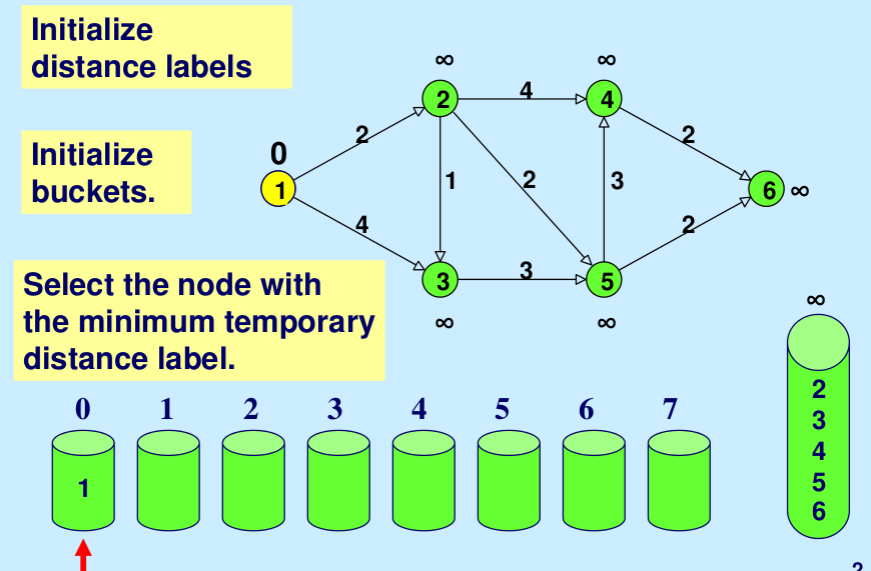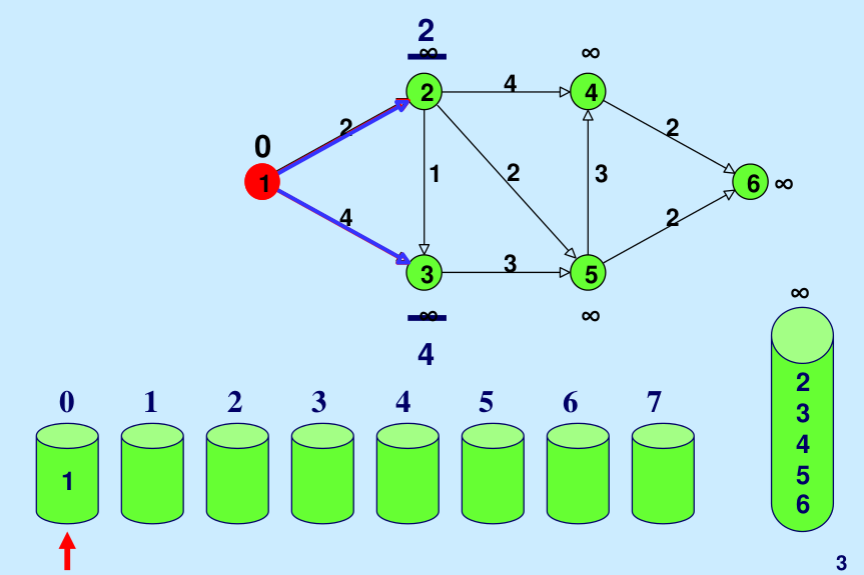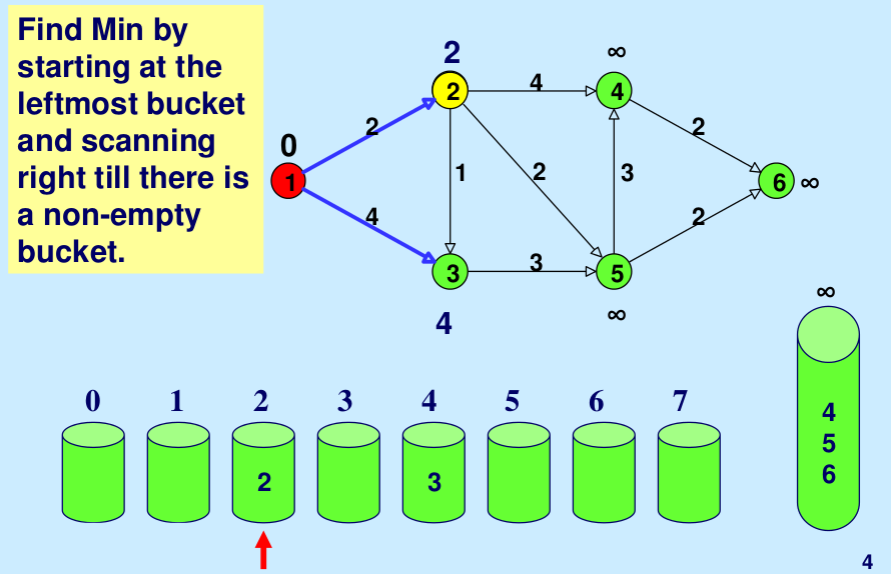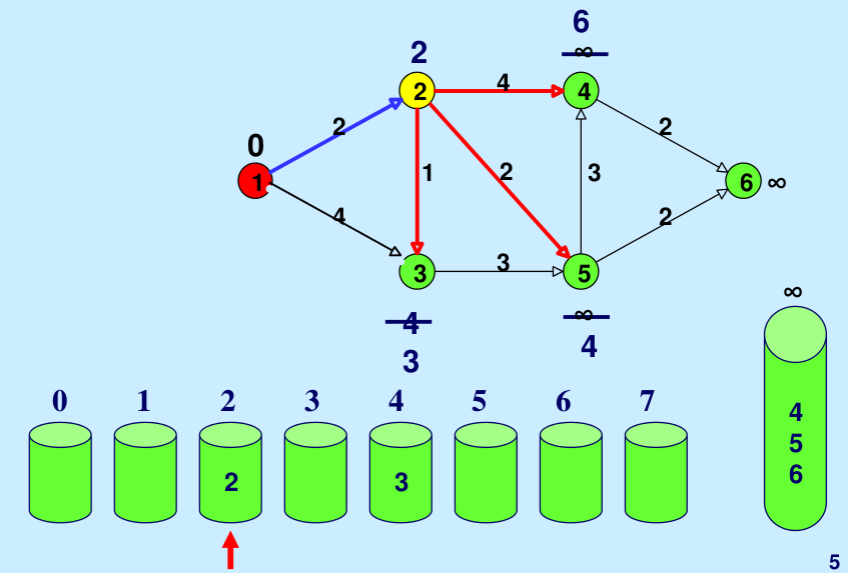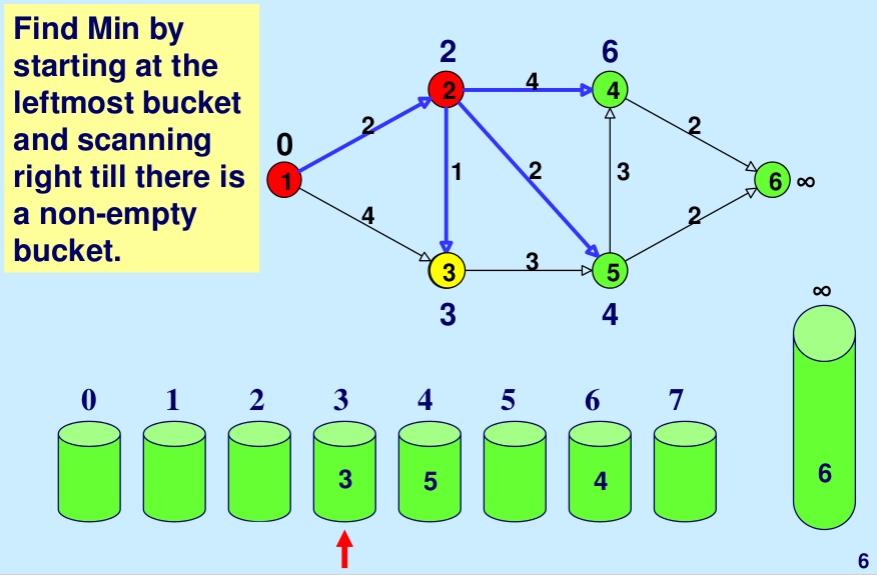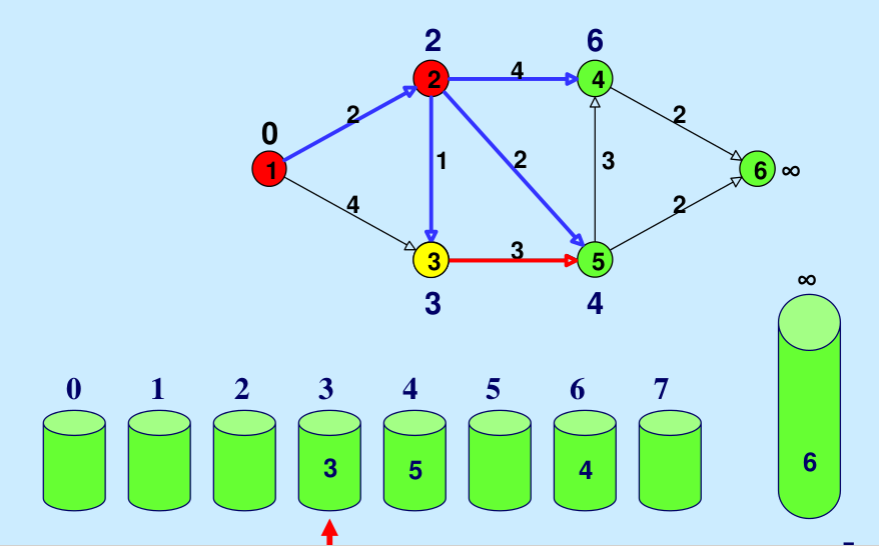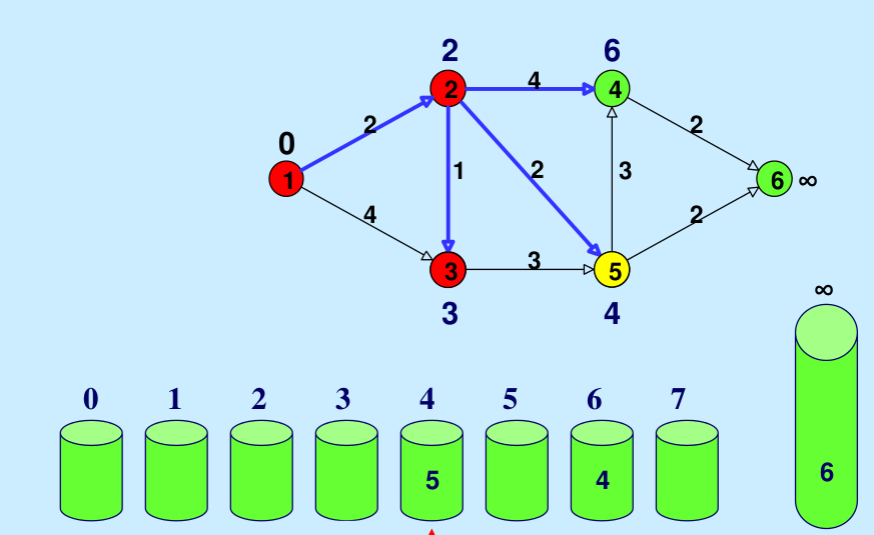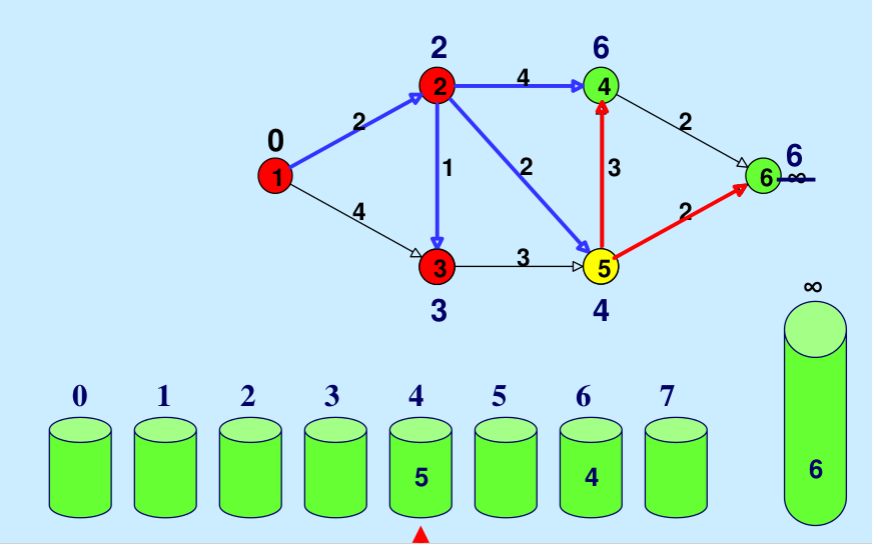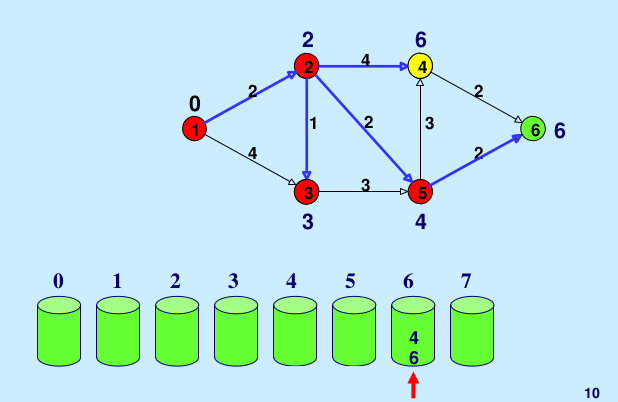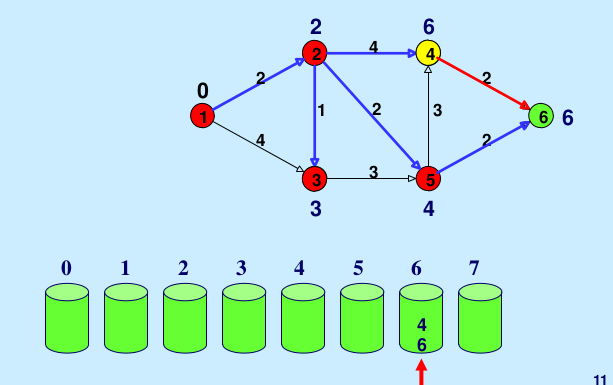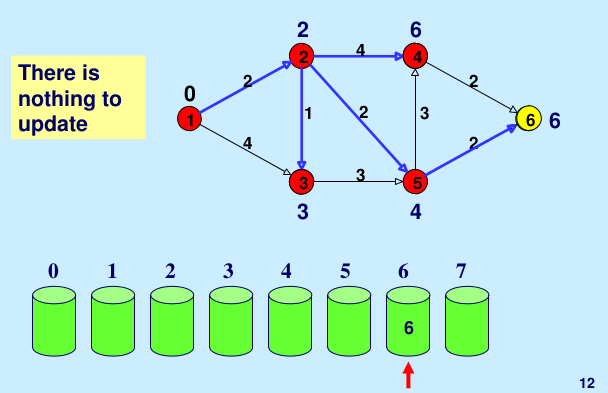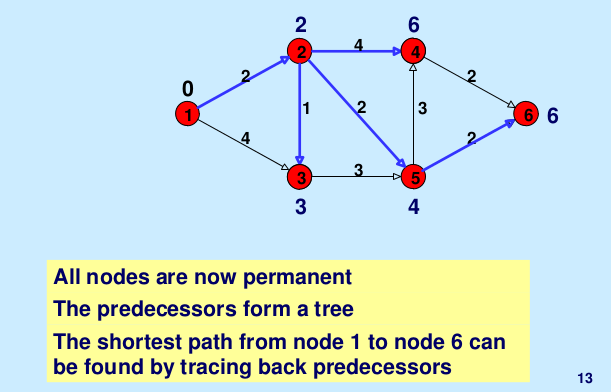Attention reader! Don’t stop learning now. Get hold of all the important DSA concepts with the DSA Self Paced Course at a student-friendly price and become industry ready.

My Personal Notes arrow_drop_up
Article Tags :
Practice Tags :

4

Please write to us at contribute@geeksforgeeks.org to report any issue with the above content.